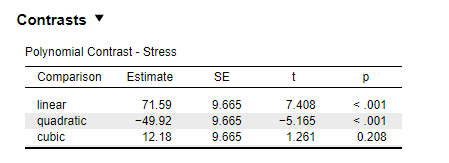#### Howdy, Stranger!

It looks like you're new here. If you want to get involved, click one of these buttons!

Supported by

# Divergence between JASP and SPSS ouptuts when computing polynomial contrasts for within-sjts designs

edited February 21

Hi,
I am trying to reproduce David Howell's SPSS analysis of the aiport dataset (attached) in JASP. Dave's analysis is available at https://www.uvm.edu/~dhowell/StatPages/More_Stuff/RepMeasMultComp/RepMeasMultComp.html. When performing the polynomial contrast analysis of the effect of time on stress level (condition near aiport only), SPSS returns F(1, 99) = 1.721 p = .193 for the cubic trend while JASP returns t = 1.261 p = .208:Obviously the t statistic returned by JASP is not equal to the square root of F returned by SPSS. Could you please clarify why?
Best,
Mat

## Comments

• Hi Mat,
I just ran the analysis in R to check those results. Here is the code I wrote:

``````myDat <- read.csv("/home/johnny/Downloads/airport.csv")

# Take subset for "Near" condition
myDat <- subset(myDat, LOCATION == 1)

# Turn data frame into the "long data" format
myDat <- data.frame(y = unname(unlist(myDat[,1:4])),
x = as.factor(rep(1:4, each = 100)),
ID = as.factor(rep(1:100, 4)))

# Set contrast
contrasts(myDat\$x) <- contr.poly(4)

# Run RM ANOVA
myAOV <- aov(y ~ x + Error(ID/x), data=myDat)

# extract relevant contrasts from AOV object
summary(myAOV, split=list(x=list("Linear"=1, "Quad"=2, "Cubic"=3)))
# Returns
#               Df  Sum Sq Mean Sq F value   Pr(>F)
# x: Cubic    1   14843   14843   1.589    0.208
``````

So this is the same result as JASP provides.
I did some digging into how these discrepancies arise, and found this post on StackExchange that provides some great insight:
``While the "t-test approach" tests each contrast against its own separate error term, the RM-ANOVA tests each contrast against a pooled error term (hence DF=14), which assumes that the variances of the contrast scores are approximately equal (i.e., sphericity assumption)."
It seems SPSS has the "t-test approach" without the pooled error term, and JASP/aov have the approach with the pooled error term. We have recently implemented the option to switch between pooled/nonpooled error terms for the post-hoc tests, and I do think it's prudent to add the same option to contrasts for those cases where the sphericity assumption has been violated. Thanks for pointing this out to us!
Kind regards,
Johnny

Thanked by 1mservant
Sign In or Register to comment.